## General Question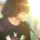# Do you ever need to use imaginary numbers in real life?

Asked by applesaucemanny (1775) June 26th, 2009

I understand how we use log and scientific notation and all that but when are we going to need to use imaginary numbers?

Observing members: 0Composing members: 0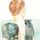Ah, “i.” As far as I am aware, imaginary numbers are only used by some computer/other technology-related occupations and mathematicians.
Isn’t math fun?

Fly (8726)“Great Answer” (0) Flag as…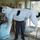When I taught control theory, electromagnetism, quantum mechanics, and cartography I used them quite often. not so much anymore.

Yes, math is fun…

DrBill (16056)“Great Answer” (3) Flag as…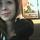I love the number elevendy. I use it all the time. Or elevendy billion. :]

chelseababyy (7934)“Great Answer” (2) Flag as…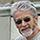No, my imaginary friend uses them – but I abstain.

DarkScribe (15480)“Great Answer” (6) Flag as…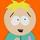How many times has a question about gay rights and marriage been asked on Fluther? Better go with the N times.

filmfann (47299)“Great Answer” (0) Flag as…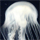When boredom strikes.

Dum dum da dum..

Saturated_Brain (5235)“Great Answer” (0) Flag as…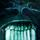Only when discussing my bank balance.

Lightlyseared (31819)“Great Answer” (1) Flag as…@Lightlyseared so… how many i’s do you have?

applesaucemanny (1775)“Great Answer” (0) Flag as…1. Just after the L.

Lightlyseared (31819)“Great Answer” (1) Flag as…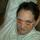What the hell is an imaginary number?

knitfroggy (8962)“Great Answer” (0) Flag as…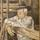They are very useful on tax returns.

juwhite1 (2971)“Great Answer” (1) Flag as…You may never be taught this, but here is a neat application of imaginary numbers to trigonometry. It can be used to find multiple angle formulas.

Suppose you wanted to know how to find cos(3x) in terms of powers of cos(x) and sin(x)

cos(3x) + isin(3x) = e^(3ix) = (e^ix)^3 = (cos(x) + isin(x))^3
You would now expand the right side. The real part of what you get is cos(3x) and the imaginary part is sin(3x)

You can also go in the opposite direction. Suppose you wanted to find cos^3(x) in terms of sin and cos of multiples of x.
cos^3(x) = [(e^ix – e^-ix)/2]^3 Expand the right side, giving powers of e^ix. Then convert these terms to sin and cos of multiples of x. The imaginary terms will cancel and you end up with the desired equation.

LostInParadise (27413)“Great Answer” (1) Flag as…I saw the cos(3x) on the third line and blanked out. It’s amazing, I can actually feel my brain forcing my eyes away from the equation while refusing to even try comprehending what’s going on…

Saturated_Brain (5235)“Great Answer” (2) Flag as…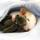i do.

ratboy (15157)“Great Answer” (1) Flag as…@ratboy So punny!

Fly (8726)“Great Answer” (0) Flag as…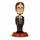Electrical analysis requires you to use them.

critter1982 (4120)“Great Answer” (0) Flag as…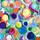I don’t know how anything remotely math related made it into my “Questions for you.” Am I being punished?

ubersiren (15165)“Great Answer” (0) Flag as…@ubersiren – Must be the “imaginary” factor. ;-)

juwhite1 (2971)“Great Answer” (0) Flag as…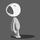This is a very good question. The simple answer is yes, if by “real life” you mean anything that is observable in the “everyday sense” kind of way. If you are a mathematician, or your field uses mathematics quite often, you use complex (imaginary) numbers regularly simply because they get the job done… and very elegantly at that.

I would say the majority of the numbers encountered by most scientists deal with rational numbers. And especially numbers that are truncated… approximations of numbers that have a huge (sometimes infinite… think pi) number of digits. The simple fact is, the entire collection of real numbers is severely neglected in everyday use. But, this is ok. They’re simply not useful in every context.

What about imaginary numbers? These beasts have a very specialized, theoretical, purpose. To mathematicians they are just another extension of the real number system (and yes, there is an infinite regress of extensions beyond complex numbers – starting with hyper-complex numbers). To scientists, they provide a very compact way to express theories of periodic (repeating, etc.) phenomena. They also produce fractals .(they are interesting and useful!)

Typically, the symbol ‘i’ is used to represent complex numbers. It is a bit unsettling, after being introduced to complex numbers, that they do not look like numbers at all. They look like algebra . Most of our entire math education has drilled into us the falsehood that numbers are only represented in one way: a bunch of numerals lined up in a row, sometimes with a decimal point in the middle of them all. No wonder our heads hurt after first seeing our first complex number, and no wonder why we see no purpose for them in everyday life (complex numbers aren’t alone in this fact). The strangeness of ‘i’ is no stranger than seeing the square root of 2 (with the radical sign over the two) ... that is just as weird, because that symbol represents exactly a number who’s square is 2. The same for ‘i’, the ‘number’ who’s square is -1.

majamin (99)“Great Answer” (1) Flag as…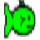Yes, in Electronics we use them all the time. sqrt(-1) is represented by the letter j, as i always represents current. In a reactive circuit – one that contains inductors, capacitors, or both, voltage and current are out of phase. The phase difference can be represented as a vector, or phasor on an Argand diagram. The Argand diagram is a coordinate system with + and – real numbers on the horizontal axis, and + and – imaginary numbers on the vertical axis. A circuit with a phase difference of +30 degrees would be represented by the phasor sqrt(3)/2+j.

What’s the significance of this? In a purely reactive circuit – one that contains only a capacitor, for instance, you can have current flowing, and a difference in voltage across the terminals, but no power dissipation. That corresponds to a phase difference of -90 degrees, which is represented on the Argand diagram as 0 – j.

In a circuit that contains both resistive and reactive components, only the resistive components dissipate power, while the reactive components dissipate nothing. Thus their power dissipation could be called imaginary. You can measure the voltage across a reactive circuit and the current through it, but you need to know the phase relationship between the voltage and current to determine how much power is actually being used.

IchtheosaurusRex (8661)“Great Answer” (0) Flag as…or